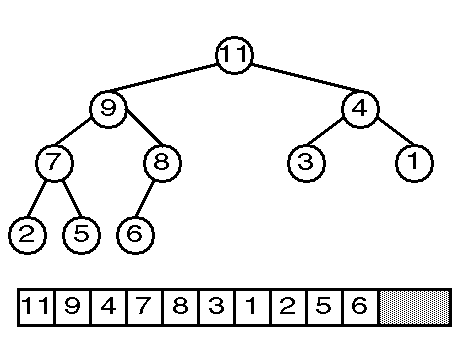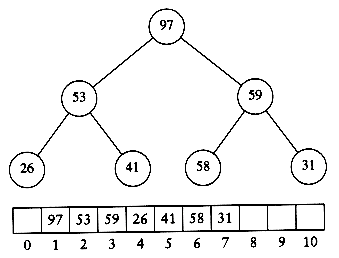## Learning Objectives

By the end of this class, you should know...
• What is a heap?
• What is the difference between a max and a min heap?
• What is the heap property of:
• a max heap?
• a min heap?
• How do you build a heap from an unordered array?
• What are the Big-O run-time of the build operation on a heap?

## 1. Heap Pre-Assessment

• Below is the representation of a heap.
• Let's get familiar with heaps and review some important concepts:
• What does it resemble?
• A tree?
• A binary tree?
• A binary search tree?
• A balanced tree?### 2.1. What Is a Heap?

• A Heap is an ADT that is used to represent a Complete Binary Tree (also called an "Almost Complete Binary Tree").
• Recall from Lesson 9 that a Complete Binary Tree is one where:
• all levels are filled (except the last)
• all nodes are as far left as possible
• For efficiency, the heap data can be stored in an array.Image source.

Note that a Heap does not satisfy the conditions for a BST.
• Instead, a heap maintains one of the Heap Properties:

### 2.2. Max Heap Property

A[Parent(i)] >= A[i]

### 2.3. Min Heap Property

A[Parent(i)]  <= A[i]

• Based on these properties, what kind of heap is the heap above: Max or Min?
• We will be working with Max Heaps in this class.

### 2.4. Additional Properties of Heaps

• The images shown above are only representations of a heap that help us to visualize its structure.
• Like a hash table, the data in a heap is stored in an array.
• However, consider that a heap is a binary tree that is stored as a linear type (an array).
• Therefore, a certain ordering must be maintained inside the array:
1. Root of the heap is at A
2. Parent of any node A[i]A[floor(i/2)]
3. left_child of A[i] = A[2i]
4. right_child A[i] = A[2i + 1]

Verify that these properties are true using the diagram below:• Node:     53        Index:

Node’s Parent:   Parent’s Index:

Node:     59        Index:

Left Child:            Left Child’s Index:

Right Child:      Right Child’s Index:

• Note that the above formulas need to be adjusted if you are indexing your array starting at 0 instead of 1.
• However, typical heap implementations will simply avoid storing any data in the 0th index of the array.

### 2.5. Applications of Heaps

• Used for Priority Queue
• A Priority Queue is similar to the FIFO Queue, but each node also stores a priority value. The first out has highest priority.
• Sorting algorithm: Heap Sort
• Will cover at the end of heap lesson
• Selection algorithms:
• Efficiently find the kth smallest (or largest) element in the array.

• Heapify
• Build Heap
• Heap-Insert
• Heap Sort

## 3. Max-Heapify

• Given a tree which is a valid max heap -- except for node i, the max-heapify algorithm will rearrange node i and its descendants to satisfy the max-heap property.
• Precondition: left(i) and right(i) are both valid max heaps
• In other words, we have one value that is out of place. We need to fix the heap!

3.1. Max-Heapify Example:

Image source.

• 4 is out of place. The subtrees at 7 and 8 are both valid max heaps.
• Swap 4 with its largest child (the right child - 8).
• Is 4 in the correct position after this swap? No.
• Swap 4 with its largest child (the left child -6).
• Is 4 in the correct position after this swap? Yes!

#### 3.2. Max-Heapify PseudoCode

Max-Heapify(A, i)

l = left(i) //get the index of the left child of A[i] and store as l

r = right(i) //get the index of the right child of A[i] and store r

//Check if l is off the end of the array (heap) AND compare A[i] to its left child

if (l <= Heap_size(A) AND A[l] > A[i])

index_of_max = l //update index_of_max if left is bigger

//Check if r is off the end of the array (heap) AND compare A[r] to current max value

if (r <= Heap_size(A) AND A[r] > A[index_of_max]

index_of_max = r //update index_of_max if right is bigger

if (i != index_of_max) //if A[i] was not bigger than its two children

A[i]<--->A[index_of_max] //swap, so now A[i] stored at A[index_of_max]

Max-Heapify(A, index_of_max) //recursively move through tree until restore heap property

## 4. Build-Heap

• This algorithm takes an un-ordered array and turns it into a valid Heap
• The build-heap algorithm starts around the middle of the array.
• It swaps nodes where the parents are smaller than the children (bubbles the values down) until the nodes have reached their correct locations.
• It uses max-heapify as a helper algorithm to achieve this bubbling down process.
• The build heap algorithm creates larger and larger valid subheaps until the entire heap is valid.

### 4.1. Build Heap Example

#### 4.2. Build-Heap Pseudocode

Build-Heap(A)

n = Heap_size(A)

for (i = floor(n/2) down to 1) //start at floor(n/2); we can ignore leaf nodes

Max-Heapify(A, i) //call Max-Heapify helper function

#### 4.3. Run-Time of Build-Heap: O(n) (surprise!)

The intuition:
• The algorithm ignores any leaf nodes (starts at index floor(n/2)).
• Works bottom up.
• Every subsequent level requires potentially more swaps,
• But for only half the number of nodes as the prior level (fewer nodes at each level as you go up the tree)Image source.

## 5. Build Heap Practice

Trace the build-heap algorithm on the given un-ordered arrays:
• Call BuildHeap on A= [11, 5, 10, 12, 9, 8, 2, 6, 2, 15]
• Call BuildHeap on A2 = [12, 7, 15, 9, 2, 4, 6, 8]

## Wrap Up

• Answer the review questions for this lesson on Canvas

~ Have a Great Day! ~